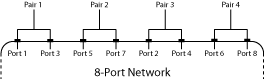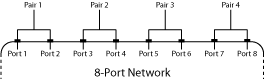# s2scc

Convert single-ended S-parameters to common-mode S-parameters (Scc)

## Syntax

``scc_params = s2scc(s_params)``
``scc_params = s2scc(s_params,option)``

## Description

example

````scc_params = s2scc(s_params)`converts the 2N-port single-ended S-parameters to N-port common-mode S-parameters. ```
````scc_params = s2scc(s_params,option)` converts S-parameters based on the port-ordering convention specified in `option` argument.```

## Examples

collapse all

Convert network data to common-mode S-Parameters.

```s_params = sparameters('default.s4p'); s4p = s_params.Parameters; s_cc = s2scc(s4p);```

To display common-mode S-Parameters at the first frequency, type the following command:

`s_cc_new = s_cc(:,:,1)`
```s_cc_new = 2×2 complex 0.1799 - 0.1839i -0.5300 - 0.6771i -0.5314 - 0.6800i 0.1756 - 0.1910i ```

## Input Arguments

collapse all

2N-port single ended S-parameters, specified as a 2N-by-2N-by-M array of complex numbers, where M represents the number of frequency points of 2N-port single-ended S-Parameters.

Port order, a scalar, specified as `1`, `2`, or `3`. Port order determines how the function orders the ports:

• `1``s2scc` pairs the odd-numbered ports together first, followed by the even-numbered ports. For example, in a single-ended, 8-port network:

• Ports 1 and 3 become common-mode pair 1.

• Ports 5 and 7 become common-mode pair 2.

• Ports 2 and 4 become common-mode pair 3.

• Ports 6 and 8 become common-mode pair 4.

The following figure illustrates this convention for an 8-port device.• `2``s2scc` pairs the input and output ports in ascending order. For example, in a single-ended, 8-port network:

• Ports 1 and 2 become common-mode pair 1.

• Ports 3 and 4 become common-mode pair 2.

• Ports 5 and 6 become common-mode pair 3.

• Ports 7 and 8 become common-mode pair 4.

The following figure illustrates this convention for an 8-port device.• `3``s2scc` pairs the input ports in ascending order and the output ports in descending order. For example, in a single-ended, 8-port network:

• Ports 1 and 2 become common-mode pair 1.

• Ports 3 and 4 become common-mode pair 2.

• Ports 8 and 7 become common-mode pair 3.

• Ports 6 and 5 become common-mode pair 4.

The following figure illustrates this convention for an 8-port device.## Output Arguments

collapse all

N-port common-mode S-parameters , returned as an N-by-N-by-M array of complex numbers, where M represents the number of frequency points of 2N-port common-mode S-parameters (Scc).

 Fan, W., et al. “Mixed-Mode S-Parameter Characterization of Differential Structures.” Proceedings of the 5th Electronics Packaging Technology Conference (EPTC 2003), IEEE, 2003, pp. 533–37. DOI.org (Crossref), doi:10.1109/EPTC.2003.1271579.